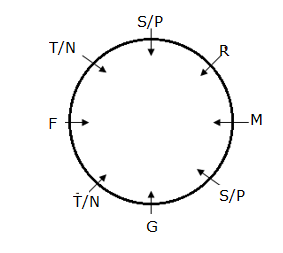# SBI Clerk Mains Reasoning (Day-05)

Dear Aspirants, Our IBPS Guide team is providing new series of Reasoning Questions for SBI Clerk Mains 2020 so the aspirants can practice it on a daily basis. These questions are framed by our skilled experts after understanding your needs thoroughly. Aspirants can practice these new series questions daily to familiarize with the exact exam pattern and make your preparation effective.

Start Quiz

Ensure Your Ability Before the Exam – Take SBI Clerk 2020 Mains Free Mock Test

Coding decoding:

(Directions 1–4): Study the following information carefully and answer the questions given below it.

Alpha-numeric elements are to be coded as follows:

Conditions:

i) If the first element is an even digit and the last element is a consonant, then both are to be coded as the code for the last element.

ii) If the second element is a consonant and the fourth element is a vowel then codes of third and fifth digits are to be interchanged.

iii) If both the first and the last element are an even digit, then both are to be coded as the code for the first element.

iv) If the first element is an odd digit and the last element is an even digit, then their codes are to be interchanged.

v) If the second element is a vowel and the fourth is a consonant, then codes of third and sixth element are to be interchanged.

1) What is the code for “6B7EYU2”?

a) *#£&¥%@

b) *£&#¥%*

c) *#&£¥%*

d) @#&£¥%*

e) None of these

2) What is the code for “5E6YJG8”?

a) €£Ω\$&*!

b) €£Ω&\$*!

c) €£Ω\$&*€

d) €£%\$&*!

e) None of these

3) What is the code for “8E5U63F”?

e) None of these

4) What is the code for “3GUE8F6”?

e) None of these

Direction sense:

Directions (5-7): Study the following information carefully and answer the questions given below:

If,

[email protected]’ means P is 2m north of Q.

‘P\$Q’ means P is 10m south of Q.

‘P&Q’ means P is 5m east of Q.

‘P#Q’ means P is 4m west of Q.

5) In which direction does T with respect to L, if the following expression is ‘T&[email protected]#[email protected]’?

a) North West

b) South East

c) North East

d) South West

e) None of these

6) How far and in which direction does A with respect to E, if the following expression is A#Z, B&A, [email protected], M\$C, such that distance between E and Z is 1m more than the distance between C and B, E is north west of C?

a) 4m, North East

b) 5m, South West

c) 5m, North West

d) Cannot be determined

e) None of these

7) If the following expression is K\$R&[email protected]#T and Q is north of K,L is west of Q, then what is the distance between Q and T?

a) 2m

b) 3m

c) 5m

d) 1m

e) None of these

Data Sufficiency :

Directions (8-10): Each of the questions below consist of a question and two statements numbered I and II given below it. You have to decide whether the data provided in the statements are sufficient to answer the question. Read both the statements and give answer

(a) if the data in statement I alone are sufficient to answer the question, while the data in statement II alone are not sufficient to answer the question.

(b) if the data in statement II alone are sufficient to answer the question, while the data in statement I alone are not sufficient to answer the question.

(c) if the data either in statement I alone or in statement II alone are sufficient to answer the question.

(d) if the data in both statement I and II together are not sufficient to answer the question.

(e) if the data in both statement I and II together are necessary to answer the question.

8) Seven persons V, W, R, X,Y, M and Z lives on eight different floors, such that lowermost floor is numbered 1, the above floor is numbered 2, and the topmost floor is numbered 7 but not necessarily in the same order. Who lives on fifth floor?

I) V lives on odd numbered floor. R lives immediately above V. As many persons lives above Y is same as below W. Y lives above W, who does not live on lowermost floor.

II) Two persons live between M and X. M does not live immediately above of W.

9) Eight persons N, F, T, S, P, G, M and R are sitting around a circular table facing centre. Who sits second to the left of M?

I) Two persons sit between G and R. F sits immediate left of the one who sits opposite to R. As many persons sit between T and F sit between F and N. Two persons sit between S and P.

II) G sits immediate left of P. Three persons sit between P and T. S sits immediate right of R.

10)  What is the code for ‘fight’?

I) ‘Minority poor marginalised’ is coded as ‘smrq tm’ and ‘dissent policies fight is coded as ‘pslqdf’.

II) ‘dissent poor travel’ is coded as ‘psrqmn’ and ‘number of policies’ is coded as ‘wl ax lq’.

Directions (1-4) :

Given series = “6B7EYU2”

It follows condition (ii) & (iii), thus “6B7EYU2”can be coded as “*#&£¥%*”.

Given series = “5E6YJG8”

It follows condition (iv) & (v), thus “5E6YJG8”can be coded as “€£Ω&\$*!”

Given series = “8E5U63F”

It follows condition (i), thus “8E5U63H”can be coded as “Ψ£!%*©Ψ”.

Given series = “3GUE8F6”

It follows condition (ii)& (iv), thus “3GUE8F6”can be coded as“*Ω€£%Ψ©”.

Directions (5-7) :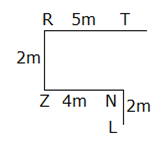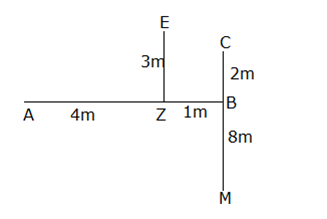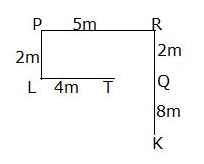Directions (8-10) :

From I and II,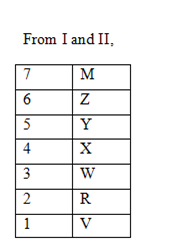From I,